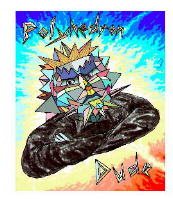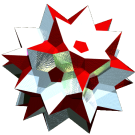Acronym so Name stella octangula,compound of 2 tet Coxeter symbol {4,3}[2{3,3}]{3,4} `© ©©` Circumradius sqrt(3/8) = 0.612372 Inradius 1/sqrt(24) = 0.204124 Vertex figure  General of army cube Colonel of regiment (is itself locally convex) Dihedral angles (at margins) between {3} and {3}:   arccos(1/3) = 70.528779° Confer related compounds: sis   general polytopal classes: regular ExternallinksThe common intersection of the stella octangula is a (scaled) oct. Moreover stella octangula is selfdual.

Aside: In projective space even a third tetrahedron could be added such, that the edges all intersect by three. In fact one vertex then would be at the center and the others at the plane of infinity. This configuration then is known as the desmic tetrahedra: a representation of a desmic configuration of 3 sets of points (here: the vertices of either tetrahedron), where any line through 2 points from 2 of these sets also passes through a point of the third set.

Incidence matrix according to Dynkin symbol

```xo3oo3ox

o.3o.3o.  & | 8 |  3 | 3 || 1
------------+---+----+---++--
x. .. ..  & | 2 | 12 | 2 || 1
------------+---+----+---++--
x.3o. ..  & | 3 |  3 | 8 || 1
------------+---+----+---++--
x.3o.3o.  & ♦ 4 |  6 | 4 || 2
```

```o3o4β

both( . . . ) | 8 |  3 | 3 || 1
--------------+---+----+---++--
both( . o4s ) | 2 | 12 | 2 || 1
--------------+---+----+---++--
sefa( o3o4β ) | 3 |  3 | 8 || 1
--------------+---+----+---++--
both( o3o4s ) ♦ 4 |  6 | 4 || 2

starting figure: o3o4x
```

```β2o4β

both( . . . ) | 8 | 2 1 | 3 || 1
--------------+---+-----+---++--
both( s 2 s ) | 2 | 8 * | 2 || 1
both( . o4s ) | 2 | * 4 | 2 || 1
--------------+---+-----+---++--
sefa( β2o4β ) | 3 | 2 1 | 8 || 1
--------------+---+-----+---++--
both( s2o4s ) ♦ 4 | 4 2 | 4 || 2

starting figure: x o4x
```

```β2β2β

both( . . . ) | 8 | 1 1 1 | 3 || 1
--------------+---+-------+---++--
both( s2s . ) | 2 | 4 * * | 2 || 1
both( s 2 s ) | 2 | * 4 * | 2 || 1
both( . s2s ) | 2 | * * 4 | 2 || 1
--------------+---+-------+---++--
sefa( β2β2β ) | 3 | 1 1 1 | 8 || 1
--------------+---+-------+---++--
both( s2s2s ) ♦ 4 | 2 2 2 | 4 || 2

starting figure: x x x
```